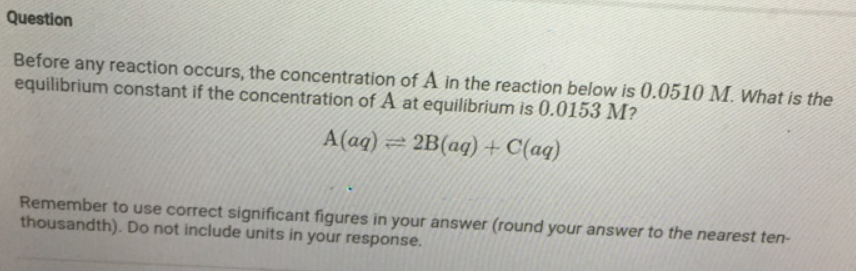# Problem: Before any reaction occurs, the concentration of A In the reaction below is 0.0510 M. What is the equilibrium constant if the concentration of A at equilibrium is 0.0153 M? A (aq) ⇌ 2B (aq) + C (aq) Remember to use correct significant figures in your answer (round your answer to the nearest ten thousandth). Do not include units in your response.

###### FREE Expert Solution
91% (159 ratings)###### Problem Details

Before any reaction occurs, the concentration of A In the reaction below is 0.0510 M. What is the equilibrium constant if the concentration of A at equilibrium is 0.0153 M?

A (aq) ⇌ 2B (aq) + C (aq)